# We Helped With This R Programming Homework: Have A Similar One?

SOLVEDCategory Programming R | R Studio Graduate Solved Statistic Help Online

## Short Assignment Requirements

This is an Assignment about Machine Learning and is intended for implementing Stochastic Gradient Descent (SGD) algorithm on given datasets using the programming language R Studio. The professor has clearly said that he wants us to implement the algorithm ourselves, that is NOT making use of built-in R functions such as "sgd(x, ...)" or any other versions.Only Task 1 and 2 are to be solved, Task 3 is NOT relevant at all (ignore please).On top of the attached Project Description in PDF called "Assignment_R", I have also attached two necessary datasets, called "Simulated_data" and "Weather_data".The professor has provided us with some preparatory R codes to get settled quicker these are called "cost.r" , "optimizers.r" and "question1.r". I could not attach them here since there is only option for 3 elements to upload. I will upload them later.The Professor added this note: "it is allowed to tune hyper-parameters other than the ones asked to be tuned in the project. If you wish to tune and change the values of additional hyper-parameters (such as epsilon for which I gave you an indicative value), this is absolutely fine and you will not be penalised. All that matters it to ensure that you tweak the hyper-parameters in order to get an (empirically) sensible parameter estimate from each stochastic optimiser."

## Assignment Description

5M project: stochastic optimization

Big data analytics

### Project outline

The purpose of this project is to learn the basics of contemporary stochastic optimization methods. You will learn how such methods operate by implementing them and by tuning their hyperparemeters. The stochastic optimization algorithms considered in the project are stochastic gradient descent (SGD), stochastic gradient descent with momentum (MSGD), stochastic gradient descent with Nesterov accelerated gradient (NAGSGD), AdaGrad, RMSProp and Adam.

Several of these stochastic optimization approaches were developed to train convolutional neural networks. For the purposes of the project, linear regression will be used instead of deep learning models. By using a familiar model such as linear regression, your focus will be placed on understanding the stochastic optimization algorithms.

Inference for linear regression coefficients via stochastic optimization will be conducted for three different datasets. The first dataset contains 1,000 simulated data points and is associated with two regression coefficients, namely the intercept and slope of a simple linear regression model. Starting with simple linear regression and simulated data, you will develop the involved stochastic optimization algorithms. Once you have implemented the stochastic optimization methods, you will use them on two real datasets. The second dataset is available on Kaggle, pertains to weather in Szegen between 2006-2016 and it contains 96,453 observations and six parameters. The third dataset is the million song dataset (MSD), which you have encountered in the first lab and is available on the UCI machine learning repository. Recall that MSD is a big dataset, containing 515,345 data points and 91 parameters, so you will have the chance to fit multiple linear regression on a big dataset using stochastic optimization.

### R code template

A template folder is available on Moodle, which will help you get started with coding. It contains three R scripts, namely cost.r, optimizers.r and question1.r.

The script cost.r provides the definition of the cost function and its gradient for linear regression. This is the only cost function needed for the project, since linear regression is the only model applied to the three datasets.

The script optimizers.r provides the R function gd() for gradient descent (GD). You can use the gd() function for answering the relevant questions of the project and as a coding basis for developing the stochastic optimization algorithms. Moreover, optimizers.r contains R comments with function signatures for the required stochastic algorithms. You will need to complete the function body of these functions to tackle the project.

The script question1.r sources cost.r and optimizers.r initially, and provides a template of R comments subsequently to help structure your answer to question 1. Towards the end of question1.r, commented lines give you directions towards storing the numerical and visual output of your simulations for question 1.

The template folder contains also the data files simulated data.csv, weather data.csv and uci data.csv associated with questions 1, 2 and 3, respectively.

### An introduction to stochastic optimization

This section outlines the stochastic optimization methods appearing in the project. Gradient descent (GD), which has been taught in lectures 4-5 and has been coded in lab 1, is the starting point for stochastic optimization.

Let J(θ,D) be a cost function, where θ is an nθ-length parameter vector and D a dataset of nd samples. Moreover, let ∇θJ(θ,D) be the gradient of cost function J(θ) := J(θ,D) with respect to θ. The approximation θ(k) of θ at step k of GD given the approximation θ(k−1) of θ at step k − 1 is defined as

θ(k) := θ(k−1) aθJ(θ(k−1),D),                                                               (1)

where the learning rate a is a positive real number.

In the case of a linear regression model, the data are set to D := {X,y}, where X is the nd · nθ design matrix and y is the nd-length output vector associated with linear regression.

For a big data set D consisting of a large number nd of data points, the evaluation of the gradient ∇θJ(θ,D) can be computationally expensive. To reduce the computational complexity of GD, stochastic gradient descent (SGD) samples an s-size subset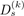of the data D at step k and evaluates the gradient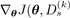) using the subset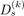. Thus, the approximation θ(k) of θ at step k of SGD given the approximation θ(k−1) of θ at step k − 1 is given by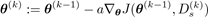.                                                     (2)

SGD is more computationally efficient than GD in large-scale machine learning problems entailing large nd or large nθ.

As an implementation hint, use the sample() function in R to sample s out of nd samples at step k without replacement. Subsequently, make sure that you index the design matrix X and output variable y appropriately.

The rest of stochastic optimization methods of this project also use a random subset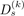of the data D at step k.

#### SGD with momentum (MSGD)

In SGD with momentum (MSGD), a ‘momentum’ term m is defined, which is a moving average of the gradient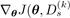), and the parameters are then updated using the momentum m instead of the gradient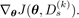The momentum m(k) and parameter approximation θ(k) at the k-th step of MSGD are defined recursively according to

m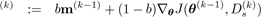,              (3) θ(k)      := θ(k−1) am(k).   (4)

MSGD has two hyper-parameters, a momentum memory factor b with values in (0,1) and a positive learning rate a. Furthermore, an initial momentum value m(0) is required by equation (3) at step k = 1.

For values of b closer to one, the moving average equation (3) retains stronger memory of momentum history. For values of b closer to zero, the moving average equation (3) remembers less any past momentum history and places more emphasis on the current gradient of the cost function.

Variant representations of MSGD are available in the literature. Equations (3)-(4) have been preferred due to providing a clearer understanding of MSGD.

#### SGD with Nesterov accelerated gradient (NAGSGD)

SGD with Nesterov accelerated gradient (NAGSGD) differs from MSGD only in the way it calculates the gradient of the cost function in the moving average equation. More specifically, NAGSGD calculates the gradient of the cost function with respect to approximate future position θ(k−1) abm(k−1) rather than with respect to the current parameter approximation θ(k−1).

Thus, step k of NAGSGD is defined as

m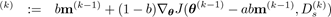, (5) θ(k) := θ(k−1) am(k).               (6)

Alternative expressions for NAGSGD can be found in the literature. Equations (5)-(6) have been chosen for the purpose of communicating the moving average model for momentum and the resulting parameter update clearly.

The learning rate a of SGD can be too small for one parameter and too large for another one. This problem tends to manifest itself for large number nθ of parameters. AdaGrad mitigates such a problem by scaling a different learning rate for each parameter adaptively and automatically.

Using the shorthand g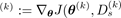), consider the outer product g(k) × g(k) at step k, which is an nθ · nθ matrix. Summing over all steps up to k yields the nθ · nθ matrix

k

G(k) := ∑g() × g().                                                                        (7)

=0

θ(k) := θ(k−1) a(diag(G(k−1)) + ϵ)⊙(−0.5) g(k−1),                                             (8)

where a is a positive learning rate, diag(G(k−1)) is the diagonal of matrix G(k−1), ϵ is a positive smoothing term that avoids division by zero (usually on the order of 1e − 8) and ⊙ denotes Hadamard (element-wise) operations. Step k = 1 requires an initial value G(0).

To further clarify AdaGrad, equation (8) will be written below in an alternative form by using element-wise notation instead of matrix notation. Let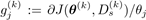be the j-th partial derivative of the cost function at step k, i.e. the j-th coordinate of the gradient ∇θJ(θ(k),Ds(k)). The AdaGrad update of the j-th parameter θj(k) at step k is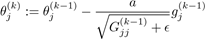,                                                      (9)

where Gjj(k−1) is the (j,j)-th diagonal element of G(k−1).Equation (9) makes it clear that AdaGrad uses a differently scaled learning rate a/Gjj(k−1) + ϵ for each parameter θj.

As a hint to assist implementation, you may focus on equation (9). You do not need to compute the outer products appearing in equation (8). It suffices to calculate the diagonal of matrix G(k), which means that it suffices to calculate the sum of squared gradients

k

Gjj(k) = ∑(gj())2.                                                                        (10)

=0

AdaGrad eliminates the need to manually tune the learning rate, since it tunes adaptively the learning rate for each parameter according to equation (9). However, the adaptive tuning of learning rates in AdaGrad comes with a weakness. Squared gradients accumulate in thedenominator of learning rate a/Gjj(k−1) + ϵ, hence learning rates shrink during training and AdaGrad is no longer able to perform parameter updates via equation (9).

#### RMSProp

RMSProp attempts to alleviate the aforementioned weakness of AdaGrad by applying a moving average model to squared gradients and by then using the output of the moving average model in the AdaGrad parameter update mechanism.

More concretely, the j-th coordinate vj(k) of the moving average of squared gradients and j-th parameterat step k of RMSprop are updated according to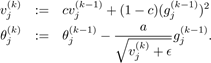,                                                   (11)

(12)

The hyperparameter c is known as the memory factor for squared gradients, taking values in c ∈ (0,1). Equation (11) requires an initial value vj(0) at step k = 1. Taking into account all coordinates j = 1,2,...,nθ, the initial value v(0) for the moving average model of RMSProp is an nθ-length vector.

Adaptive moment estimation (Adam) is a stochastic optimization method that computes a different learning rate for each parameter adaptively. Apart from retaining a moving average of squared gradients similarly to RMSProp, Adam stores a moving average of gradients additionally.

(k)

The Adam update of the j-th parameter θj              at step k is given by(13)

,                                                  (14)

(15)

(16)

(17)

Adam has three hyperparameters, namely a positive learning rate a, a memory factor b of gradients with values in (0,1) and a memory factor c of squared gradients with values in (0,1). Morevover, Adam requires the initial values m(0) and v(0) at step k = 1.

Equations (13) and (14) provide the moving average of gradients and of squared gradients, respectively. Adam takes its name after these first and second order estimators of gradients. Due to m(0) and v(0) being initialized typically as vectors of zeros, the moving averages m(k) and v(k) are asymptotically biased towards zero, especially when b and c are set to a value close to one. To contouract such biases while estimating the moving averages of gradients and of squared gradients, equations (15) and (16) are introduced.

### Question 1

The goal of question 1 is to develop the stochastic optimizers of this project using a simulated dataset and a cost function for simple linear regression.

To help you get started, the provided template folder contains an optimizers.r file with R code for gradient descent, a cost.r file with R code for the cost function for linear regression and its gradient, a question1.r file with some preliminary R code towards answering question 1 and the simulated data.csv file on which simple linear regression will be fitted.

As part of your answer, you will edit the optimizers.r file to include in it your R code for the optimizers of this project and you will also edit the file question1.r to include in it your R code for running the optimizers and simulations of question 1. You will not provide any output files or a report as part of your project submission.

Make sure that every optimizer in optimizers.r is a standalone R function with a return statement return(list(theta=theta, cost=cost))

as in the provided gd() function, to store the iterations of the parameter estimates and of the cost function.

You need to make sure before submission that the R command source("question1.r") runs successfully and stores the required results in output files as explained further below. question1.r starts with the lines

source("optimizers.r") source("cost.r")

to load the R code for the cost function for linear regression and its gradient as well as the R code for the optimizers. The subsequent line in question1.r loads the data for question 1: simulated_data <- read.csv("simulated_data.csv", header=TRUE)

simulated data.csv consists of two columns; the first column is labelled as y and represents the output variable, while the second column is labelled as covariates and plays the role of covariate. The remaining lines in question1.r are R comments meant to guide you towards answering question 1.

Firstly, standardize the covariate and create the design matrix X including a first column of ones for intercept.

Fit a simple linear regression to the data using the standardized covariate and the lm() function in R. The model will have two parameters, namely a regression coefficient corresponding to covariate and an intercept.

Use the same initial value θ(0) = (7,−8)T for all optimizers.

Run 100 iterations of GD tuning the learning rate a of equation (1) to converge towards the regression coefficient estimates obtained via lm().

Run 100 iterations of SGD by sampling a subset of s = 100 data points per iteration. Tune the learning rate a of equation (2) to converge towards the regression coefficient estimates obtained via lm(). Notice that even for such a simple example, SGD obtains an approximation to the regression coefficient estimates known from lm(). Although SGD does not yield an exact solution to the optimization problem, it provides a reasonable approximation.

Run 100 iterations of MSGD by sampling a subset of s = 100 data points per iteration. Set the initial value for momentum to be a vector of zeros, that is set m(0) = (0,0)T. Tune the learning rate a of equation (4) and memory factor b of equation (3) to converge towards the regression coefficient estimates obtained via lm().

Run 100 iterations of NAGSGD by sampling a subset of s = 100 data points per iteration. Set the initial value for momentum to be a vector of zeros, that is set m(0) = (0,0)T. Tune the learning rate a of equation (6) and memory factor b of equation (5) to converge towards the regression coefficient estimates obtained via lm().

Run 100 iterations of AdaGrad by sampling a subset of s = 100 data points per iteration. Set diag(G(0)) = (0,0)T and ϵ = 1e − 8. Tune the learning rate a of equation (9) to converge towards the regression coefficient estimates obtained via lm().

Run 100 iterations of RMSProp by sampling a subset of s = 100 data points per iteration. Set v(0) = (0,0)T and ϵ = 1e − 8. Tune the learning rate a of equation (12) and memory factor c of equation (11) to converge towards the regression coefficient estimates obtained via lm().

Run 100 iterations of Adam by sampling a subset of s = 100 data points per iteration. Set m(0) = (0,0)T, v(0) = (0,0)T and ϵ = 1e−8. Tune the learning rate a of equation (17), memory factor b of equation (13) and memory factor c of equation (14) to converge towards the regression coefficient estimates obtained via lm().

Your code in question1.r will store numerical summaries in CSV files and visual summaries in PDF files as follows:

(a)    Save the design matrix X in the comma-separated value file answer1a.csv.

(b)    Save the parameter estimates obtained via lm() and the final parameter estimates at the last iteration of each optimizer in the comma-separated value file answer1b.csv. The parameter estimates saved in answer1b.csv correspond to a 2 · 8 matrix, in which the first and second row represent the respective regression coefficient estimates θ0 and θ1, while the eight columns represent the parameter estimates obtained via lm(), GD, SGD, MSGD, NAGSGD, AdaGrad, RMSProp and Adam. R code in the form of comments has been provided in question1.r as guidance to facilitate the process of saving all parameter estimates.

(c)    Save the value of the cost function at the last itration of each optimizer in the commaseparated value file answer1c.csv. The cost function values saved in answer1c.csv correspond to a 1·7 matrix, in which the the seven columns represent the parameter estimates obtained via GD, SGD, MSGD, NAGSGD, AdaGrad, RMSProp and Adam. R code in the form of comments has been provided in question1.r as guidance to facilitate the process of saving all cost function values.

(d)   Plot the cost function of NAGSGD and of AdaGrad against the number of iterations in a single plot. Ensure that it is possible to distinguish between the overlaid cost function values of NAGSGD and of AdaGrad. Add a legend to the plot to make such a distinction clearer. Save the plot in the PDF file answer1d.pdf. R code in the form of comments has been provided in question1.r as guidance to facilitate the process of saving the plot of cost function values.

(e)    Plot the successive iterations of parameter θ0 against the successive iterations of parameter θ1 for GD and RMSProp. Moreover, add a point in the plot indicating the parameter estimate obtained via lm(). All optimizers started from the same initial parameter value θ(0) = (7,−8)T, so the trajectories of GD and RMSProp must have the same starting point in the plot. Moreover, the end points of the trajectories of GD and RMSProp must be in near proximity to the single point representing the parameter estimate obtained via lm() if the optimizers were tuned appropriately. Ensure that it is possible to distinguish between the overlaid trajectories of GD and RMSProp. Add a legend to the plot to make such a distinction clearer. Save the plot in the PDF file answer1e.pdf. R code in the form of comments has been provided in question1.r as guidance to facilitate the process of saving the plot of parameter trajectories.

Upload only the R scripts cost.r, optimizers.r and question1.r. Make sure that the R command source("question1.r") works free from bugs and that it generates the output files answer1a.csv, answer1b.csv, answer1c.csv, answer1d.pdf and answer1e.pdf. However, do not upload the output files themselves. I will assess your work by running the R command source("question1.r") and by looking into the generated output files.

### Question 2

The goal of question 2 is to run the stochastic optimizers of this project to fit multiple linear regression on a real dataset of relatively small size. Given that you have already developed the R code for stochastic optimization to tackle question 1, the only additional work required for tackling question 2 is to tune the hyperparameters of the optimizers.

The files cost.r and optimizers.r should be available after having answered question 1. Create an R script with name question2.r to include in it your R code for running the optimizers and simulations of question 2. You will not provide any output files or a report as part of your project submission.

You need to make sure before submission that the R command source("question2.r") runs successfully and stores the required results in output files as explained further below.

source("optimizers.r") source("cost.r")

to load the R code for the cost function for linear regression and its gradient as well as the R code for the optimizers. All subsequent R code for answering question 2 should be saved in question2.r.

Start by loading the data file weather data.csv, whose first line is the header containing column labels. For the purposes of this project, it suffices to know that the column labelled apparent temperature is the output variable, while the columns labelled temperature, humidity, wind speed, wind bearing and pressure will be used as covariates in the multiple linear regression model.

The dataset weather data.csv can be found on Kaggle at

https://www.kaggle.com/budincsevity/szeged-weather

There is no need to use Kaggle in order to address question 2. If you decide to look up the dataset on Kaggle, keep in mind that the original column labels have been altered to make R coding more user-friendly.

Firstly, standardize all five covariates and create the design matrix X including a first column of ones for intercept.

Fit a multiple linear regression to the data using the standardized covariates and the lm() function in R. The model will have six parameters, namely five regression coefficient corresponding to the covariates and an intercept.

Use the same initial value θ(0) = (−5,−3,4,1,10,−9)T for all optimizers.

Run 70 iterations of GD tuning the learning rate a of equation (1) to converge towards the regression coefficient estimates obtained via lm().

Run 200 iterations of SGD by sampling a subset of s = 1000 data points per iteration. Tune the learning rate a of equation (2) to converge towards the regression coefficient estimates obtained via lm(). Notice that even for such a simple example, SGD obtains an approximation to the regression coefficient estimates known from lm(). Although SGD does not yield an exact solution to the optimization problem, it provides a reasonable approximation.

Run 200 iterations of MSGD by sampling a subset of s = 1000 data points per iteration. Set the initial value for momentum to be a vector of zeros, that is set m(0) = (0,0,0,0,0,0)T. Tune the learning rate a of equation (4) and memory factor b of equation (3) to converge towards the regression coefficient estimates obtained via lm().

Run 200 iterations of NAGSGD by sampling a subset of s = 1000 data points per iteration. Set the initial value for momentum to be a vector of zeros, that is set m(0) = (0,0,0,0,0,0)T. Tune the learning rate a of equation (6) and memory factor b of equation (5) to converge towards the regression coefficient estimates obtained via lm().

Run 200 iterations of AdaGrad by sampling a subset of s = 1000 data points per iteration. Set diag(G(0)) = (0,0,0,0,0,0)T and ϵ = 1e − 8. Tune the learning rate a of equation (9) to converge towards the regression coefficient estimates obtained via lm().

Run 200 iterations of RMSProp by sampling a subset of s = 1000 data points per iteration. Set v(0) = (0,0,0,0,0,0)T and ϵ = 1e−8. Tune the learning rate a of equation (12) and memory factor c of equation (11) to converge towards the regression coefficient estimates obtained via lm().

Run 200 iterations of Adam by sampling a subset of s = 1000 data points per iteration. Set m(0) = (0,0,0,0,0,0)T, v(0) = (0,0,0,0,0,0)T and ϵ = 1e − 8. Tune the learning rate a of equation (17), memory factor b of equation (13) and memory factor c of equation (14) to converge towards the regression coefficient estimates obtained via lm().

Your code in question2.r will store numerical summaries in CSV files and visual summaries in PDF files as follows:

(a)    Save the design matrix X in the comma-separated value file answer2a.csv.

(b)    Save the parameter estimates obtained via lm() and the final parameter estimates at the last iteration of each optimizer in the comma-separated value file answer2b.csv. The parameter estimates saved in answer2b.csv correspond to a 6 · 8 matrix, in which each row represents one of the six regression coefficient estimates, while the eight columns represent the parameter estimates obtained via lm(), GD, SGD, MSGD, NAGSGD, AdaGrad, RMSProp and Adam.

(c)    Save the value of the cost function at the last itration of each optimizer in the commaseparated value file answer2c.csv. The cost function values saved in answer2c.csv correspond to a 1·7 matrix, in which the the seven columns represent the parameter estimates obtained via GD, SGD, MSGD, NAGSGD, AdaGrad, RMSProp and Adam.

(d)   Plot the cost function of NAGSGD and of AdaGrad against the number of iterations in a single plot. Ensure that it is possible to distinguish between the overlaid cost function values of NAGSGD and of AdaGrad. Add a legend to the plot to make such a distinction clearer. Save the plot in the PDF file answer2d.pdf.

Upload only the R scripts cost.r, optimizers.r and question2.r. Make sure that the R command source("question2.r") works free from bugs and that it generates the output files answer2a.csv, answer2b.csv, answer2c.csv, answer2d.pdf and answer2e.pdf. However, do not upload the output files themselves. I will assess your work by running the R command source("question2.r") and by looking into the generated output files.

### Question 3 (not assessed)

Question 3 will not be assessed, it is provided for educational purposes. If you do not tackle question 3, you will not be penalized. However, it is advised that you try to answer question 3 in order to experience the computational advantage of stochastic optimization over optimization in the case of big datasets.

The goal of question 3 is to run the stochastic optimizers of this project to fit multiple linear regression on a relativey big dataset. Given that you have already developed the R code for stochastic optimization to tackle question 1, the only additional work required for tackling question 3 is to handle data processing efficiently and to tune the hyperparameters of the optimizers.

The dataset uci data.csv used in question 3 is the same subset of the million song data set used in lab 1. It contains 515,345 songs (file rows) and 91 columns. The first column is the year of release, ranging from 1922 to 2011, which plays the role of output variable. The remaining 90 columns are continuous predictors of the year of release. uci data.csv is included in the template folder. More information about this subset of the million song data set is available at

http://archive.ics.uci.edu/ml/datasets/YearPredictionMSD

You will observe the computational cost of fine-tuning optimization given a relatively big dataset. At the same time, you will see how stochastic optimization reduces runtime dramatically in comparison to gradient descent. Moreover, you will see that stochastic optimization attains reasonable parameter estimates in a problem somewhat less trivial in terms of data space and parameter space dimensionality (nd = 515,345 and nθ = 91).

The files cost.r and optimizers.r should be available after having answered question 1. Create an R script with name question3.r to include in it your R code for running the optimizers and simulations of question 3.

The R command source("question3.r") will store the results in output files as explained further below. The anticipated runtime of the R command source("question3.r") is not more than fifteen minutes on a computer at one of the labs of the Mathematics and Statistics building.

## Assignment Code

``````

gd <- function(f, grad, y, X, theta0, npars, ndata, a, niters) {
theta <- matrix(data=NA, nrow=niters, ncol=npars)
cost <- vector(mode="numeric", length=niters)

theta[1, ] <- theta0
cost <- f(y, X, theta0, ndata)

for (i in 2:niters) {
theta[i, ] <- theta[i-1, ]-a*grad(y, X, theta[i-1, ], ndata)
cost[i] <- f(y, X, theta[i, ], ndata)
}

return(list(theta=theta, cost=cost))
}

# sgd <- function(f, grad, y, X, theta0, npars, ndata, a, niters, nsubsamples) {
# }

# msgd <- function(f, grad, y, X, theta0, npars, ndata, a, niters, nsubsamples, b, m0) {
# }

# nagsgd <- function(f, grad, y, X, theta0, npars, ndata, a, niters, nsubsamples, b, m0) {
# }

# }

# rmsprop <- function(f, grad, y, X, theta0, npars, ndata, a, niters, nsubsamples, c, epsilon, v0) {
# }

# adam <- function(f, grad, y, X, theta0, npars, ndata, a, niters, nsubsamples, b, c, epsilon, m0, v0) {
# }

``````

## Assignment Code

``````
### Cost functions for linear regression

## Cost function for linear regression

lmf <- function(y, X, theta, ndata) {
sum((X%*%theta-y)^2)/(2*ndata)
}

## Gradient of cost function for linear regression

lmgrad <- function(y, X, theta, ndata){
t(X)%*%(X%*%theta-y)/ndata
}

``````

Is it free to get my assignment evaluated?

Yes. No hidden fees. You pay for the solution only, and all the explanations about how to run it are included in the price. It takes up to 24 hours to get a quote from an expert. In some cases, we can help you faster if an expert is available, but you should always order in advance to avoid the risks. You can place a new order here.

How much does it cost?

The cost depends on many factors: how far away the deadline is, how hard/big the task is, if it is code only or a report, etc. We try to give rough estimates here, but it is just for orientation (in USD):

 Regular homework \$20 - \$150 Advanced homework \$100 - \$300 Group project or a report \$200 - \$500 Mid-term or final project \$200 - \$800 Live exam help \$100 - \$300 Full thesis \$1000 - \$3000

How do I pay?

Credit card or PayPal. You don't need to create/have a Payal account in order to pay by a credit card. Paypal offers you "buyer's protection" in case of any issues.

Why do I need to pay in advance?

We have no way to request money after we send you the solution. PayPal works as a middleman, which protects you in case of any disputes, so you should feel safe paying using PayPal.

Do you do essays?

No, unless it is a data analysis essay or report. This is because essays are very personal and it is easy to see when they are written by another person. This is not the case with math and programming.

Why there are no discounts?

It is because we don't want to lie - in such services no discount can be set in advance because we set the price knowing that there is a discount. For example, if we wanted to ask for \$100, we could tell that the price is \$200 and because you are special, we can do a 50% discount. It is the way all scam websites operate. We set honest prices instead, so there is no need for fake discounts.

Do you do live tutoring?

No, it is simply not how we operate. How often do you meet a great programmer who is also a great speaker? Rarely. It is why we encourage our experts to write down explanations instead of having a live call. It is often enough to get you started - analyzing and running the solutions is a big part of learning.

What happens if I am not satisfied with the solution?

Another expert will review the task, and if your claim is reasonable - we refund the payment and often block the freelancer from our platform. Because we are so harsh with our experts - the ones working with us are very trustworthy to deliver high-quality assignment solutions on time.

Customer Feedback

"Thanks for explanations after the assignment was already completed... Emily is such a nice tutor! "

Order #13073